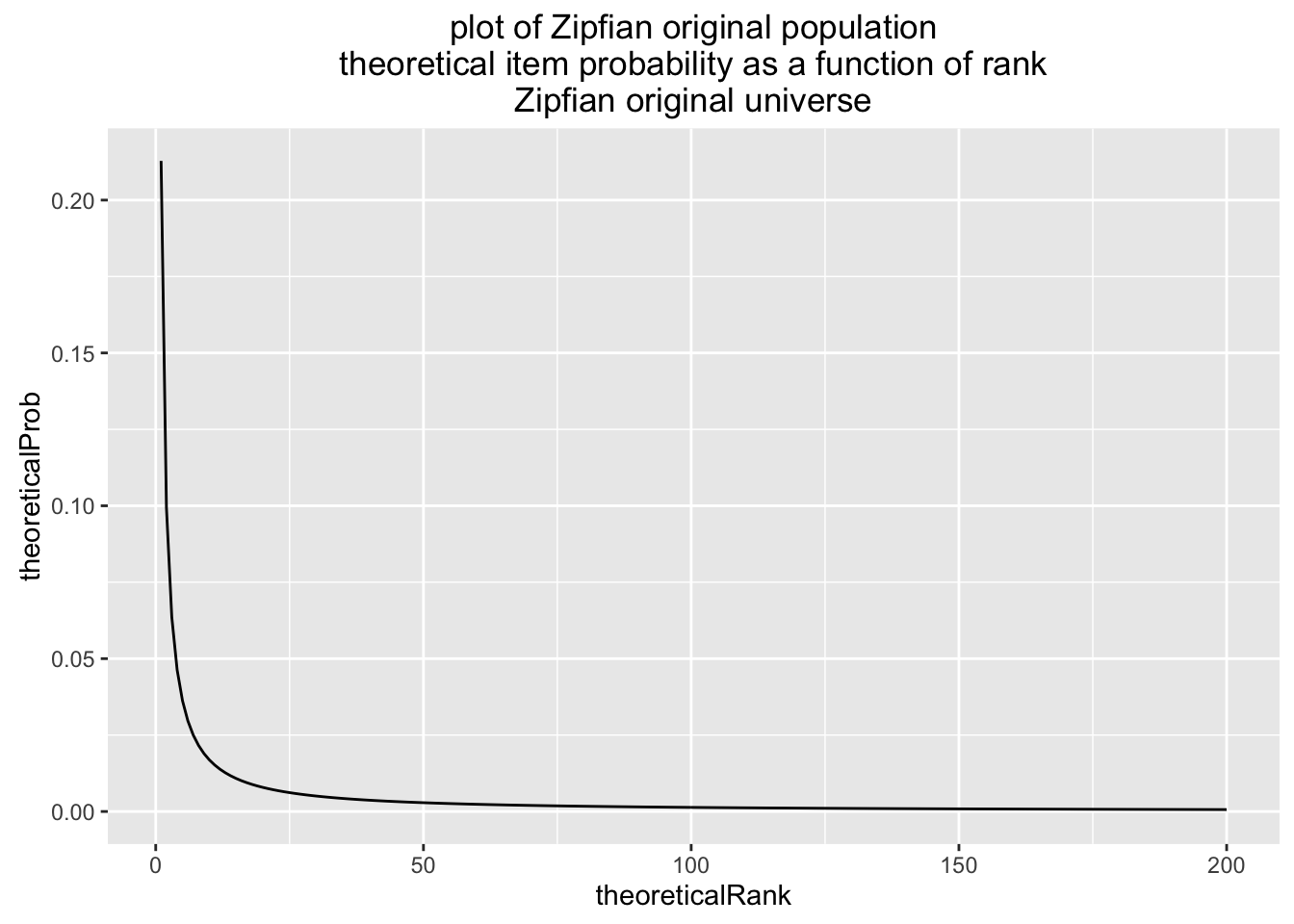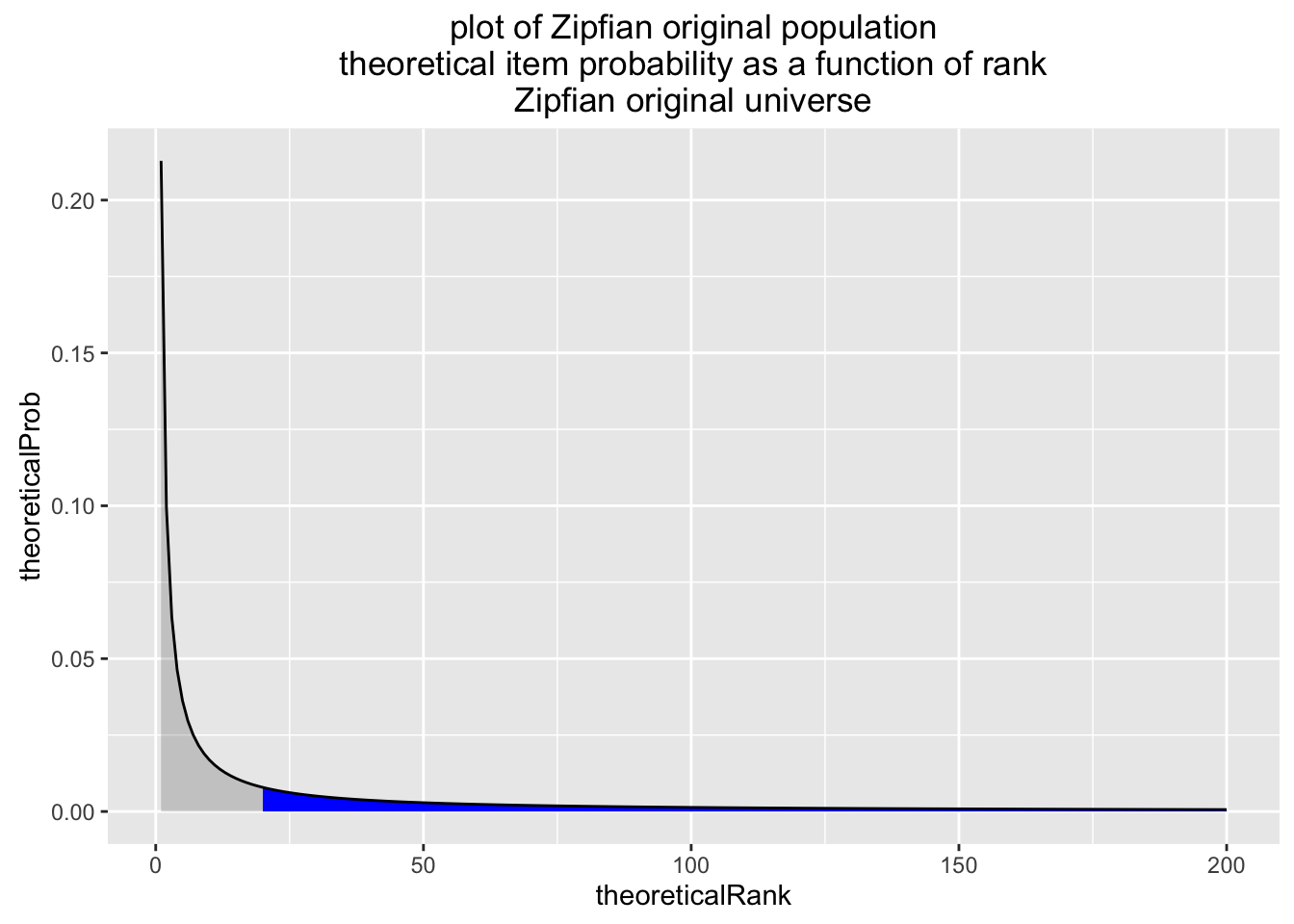Support materials for Win-Vector LLC blog article Relative error distributions, without the heavy tail theatrics.

``````knitr::opts_chunk\$set(echo = TRUE)
library('ggplot2')

# draw item i with probability pi
draw <- function(universe,n) {
u <- sort(runif(n))
idxs <- findInterval(u,c(0,universe\$cumsum),rightmost.closed = TRUE)
idxs <- pmin(idxs,nrow(universe))
# permute
idxs <- idxs[sample.int(n,n,replace=FALSE)]
idxs
}

nRanks = 200
sampleSize = 1000
contrainPosition = 20``````
``````# generate population of items with Zipfian probabilities
set.seed(23234)
zipfianUniverse <- data.frame(theoreticalRank=seq_len(nRanks))
zipfianUniverse\$theoreticalProb <- 1/zipfianUniverse\$theoreticalRank^1.1
zipfianUniverse\$theoreticalProb <- zipfianUniverse\$theoreticalProb/sum(zipfianUniverse\$theoreticalProb)
zipfianUniverse <- zipfianUniverse[order(zipfianUniverse\$theoreticalProb,decreasing=TRUE),,
drop=FALSE]
zipfianUniverse\$cumsum <- cumsum(zipfianUniverse\$theoreticalProb)

zipfianUniverse\$theoreticalProbConstrained <- zipfianUniverse\$theoreticalProb*
ifelse(zipfianUniverse\$theoreticalRank>=contrainPosition,0.01,1.0)
zipfianUniverse\$missedMass =  zipfianUniverse\$theoreticalProb -
zipfianUniverse\$theoreticalProbConstrained
zipfianUniverse\$missedMass[zipfianUniverse\$missedMass<=0] <- NA
zipfianUniverse\$theoreticalProbConstrained <- zipfianUniverse\$theoreticalProbConstrained/
sum(zipfianUniverse\$theoreticalProbConstrained)

observedZ <- data.frame(theoreticalRank=draw(zipfianUniverse,sampleSize))
countsZ <- as.data.frame(table(theoreticalRank=observedZ\$theoreticalRank))
countsZ\$theoreticalRank <- as.integer(as.character(countsZ\$theoreticalRank))
countsZ\$Freq <- countsZ\$Freq/sum(countsZ\$Freq)
countsZ\$observedRank <- rank(-countsZ\$Freq)
observedZ\$Freq <- countsZ\$Freq[match(observedZ\$theoreticalRank,
countsZ\$theoreticalRank)]

ggplot(data=zipfianUniverse,aes(x=theoreticalRank,y=theoreticalProb,
ymin=0,ymax=theoreticalProb)) +
geom_line() +
ggtitle("plot of Zipfian original population\ntheoretical item probability as a function of rank\nZipfian original universe")````````````ggplot(data=zipfianUniverse,aes(x=theoreticalRank,y=theoreticalProb,
ymin=0,ymax=theoreticalProb)) +
geom_ribbon(alpha=0.2) +
geom_ribbon(aes(ymax=missedMass),fill='blue') +
geom_line() +
ggtitle("plot of Zipfian original population\ntheoretical item probability as a function of rank\nZipfian original universe")````````sum(zipfianUniverse\$missedMass,na.rm=TRUE)``
``##  0.3254028``
``````ggplot(data=zipfianUniverse,aes(x=theoreticalRank,y=theoreticalProb)) +
geom_point() + scale_y_log10() + scale_x_log10() +
geom_smooth(method='lm',se=FALSE) +
ggtitle("log log plot of Zipfian original population\ntheoretical item probability as a function of rank\nZipfian original universe")``````